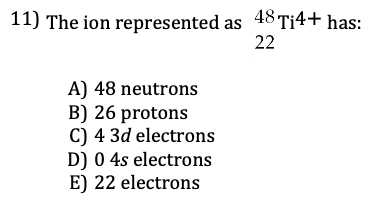##### Chemistry Chapter Two

0
Set Details Share
created 2 years ago by williammiller10
13 views
Subjects:
chemistry
Page to share:
Embed this setcancel
COPY
code changes based on your size selection
Size:
X
1

How many photons of light with frequency 5.50 × 1015 Hz are required to provide 1 kJ of energy?

a)2.74 × 10^20 photons

b)4.56 × 10^–4 photons

c) 1.65 × 10^44 photons

d) 3.64 × 10^–18 photons

e) 3.64 × 10^–16 photons

A

2

What is the energy in joules of the 656 nm spectral line of hydrogen.

a)1.45 × 10^–48 J

b) 1.30 × 10^–22 J

c) 3.03 × 10^–19 J

d) 3.03 × 10^–28 J

e) 4.35 × 10^–31 J

C

3

Calculate the wavelength, in meters, of light absorbed by an electron in an atom of hydrogen as it moves from energy level n = 3 to n = 6.

a) 8.22 × 10^–7 m

b) 1.09 × 10^–6 m

c) 3.28 × 10^–6 m

d) 1.65 × 10^–11 m

e) 1.83 × 10^–7 m

B

4

What is the wavelength of a ball that is 25.0 g traveling at 90.0 miles per hour (40.2 m/s)?

a) 1.07 × 10^–30 m

b) 2.42 × 10^–11 m

c) 6.59 × 10^–34 m

d) 6.59 × 10^–37 m

e) 4.12 × 10^–37 m

C

5

Which of the following is not possible?a) 4d

b) 2s

c) 2p

d) 5p

e) 3f

E

6

Which of the following subshells is correctly designated?

a) 3f^2

b) 4d^11

c) 2p^6

d) 3s^3

e) 1p^5

C

7

Relating Frequency and Wavelength of EM radiation: A dental hygienist uses x-rays (λ = 1.00 Ǻ) to take a series of dental radiographs. What is the frequency of the electromagnetic radiation? (assume the speed of light (c) = 3.00 × 10^8)

3.00 x 10^18 s^-1

8

The transition elements refer to what group of elements?

A) lanthanides

B) s-block elements

C) actinides

D) d-block elements

E) p-block elements

D

9

Which of the following could never be isoelectronic species?

A) atoms of two different elements

B) anions of two different elements

C) an anion and a cation

D) cations of two different elements

E) an anion and an atom

A

10D

11

What is the general valence electron configuration (where n= principal quantum number) that best describes the halogens as a group.

A) ns1np6

B) ns2np3

C) ns2np4

D) ns2np6

E) ns2np5

E

12

Which of the following has the largest atomic radius?

A) Mo

B) Br

C) I

D) Rb

D

13

A is the sequence Na, Mg, Al, Si and P. B is the sequence He, Ne, Ar, Kr, Xe. Which statement below is true?

A) The members of A are decreasing in size.

B) The members of B have larger radii than those of A, respectively. That is He > Na, Ne > Mg, etc.

C) All the members of A are isoelectronic.

D) The members of B are decreasing in size.

E) The members of A have larger radii than those of B, respectively.

A

14

Which of the following isoelectronic species has the largest radius?

A) Na+

B) Mg2+

C) F-

D) Ne

E) O2

E

15

Choose the species from which one electron could most easily be removed. A) Ca2+

B) K+

C) Ar

D) K

E) Cl

D

16

Which of the following elements is likely to have the largest second ionization energy?

A) Al

B) Mg

C) Na

D) Si

C

17

Which of the following species is diamagnetic?

A) S-

B) Al

C) C

D) F-

D

18

Which of the following has the most negative electron affinity: Li, Be, N, O, F?

A) O

B) Li

C) F

D) Be

E) N

C